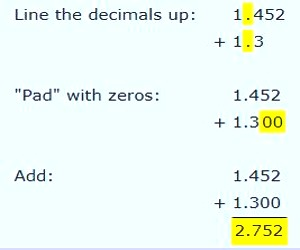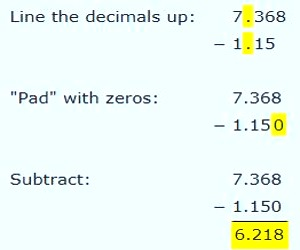Decimals are fractional numbers. The decimal 0.3 is the same as the fraction 3/10. The number 0.78 is a decimal that represents 78/100.

Always line up the decimal points when adding decimals.

Remember to put the decimal point in the proper place in your answer.

The rules of adding decimal numbers are:

(i) Write the digits of the given numbers one below the other such that all the decimal points are in the same vertical line.

(iii) Put the decimal point of the sum vertically below the other decimal points.

Example:

• Write down the numbers, one under the other, with the decimal points lined up
• Put in zeros so the numbers have the same length

Problem 1: Add 1.452 to 1.3Problem 2: Subtracting: To subtract, follow the same method: line up the decimals, then subtract.

Example: What is 7.368 − 1.15?Information Source: AAA Math